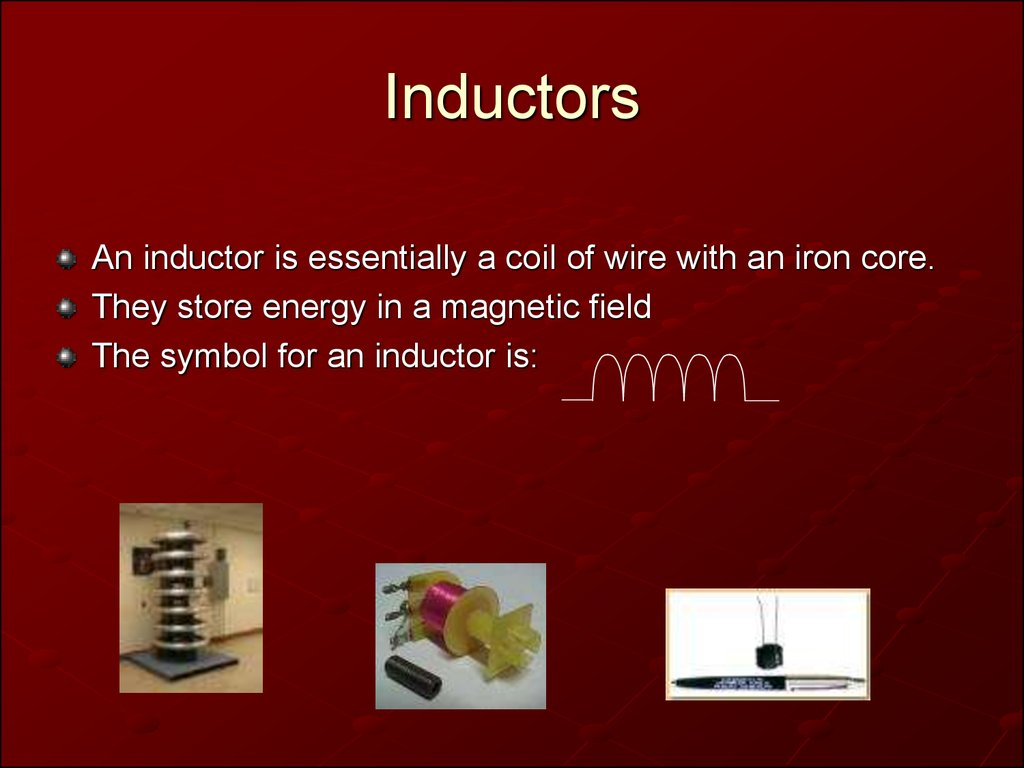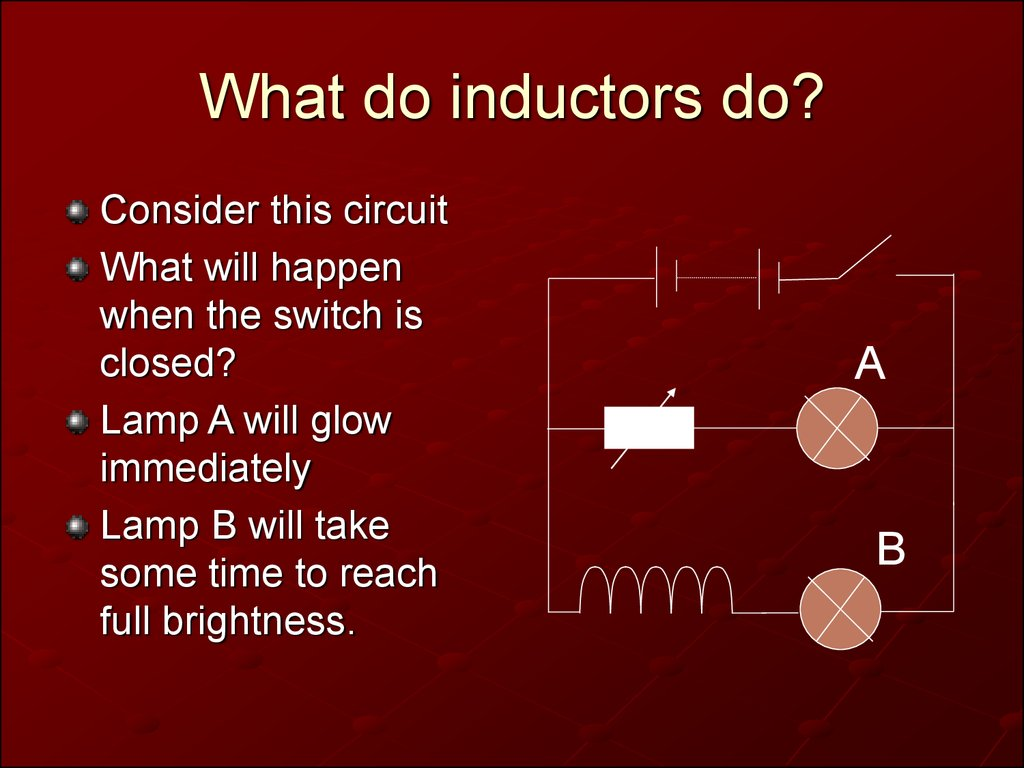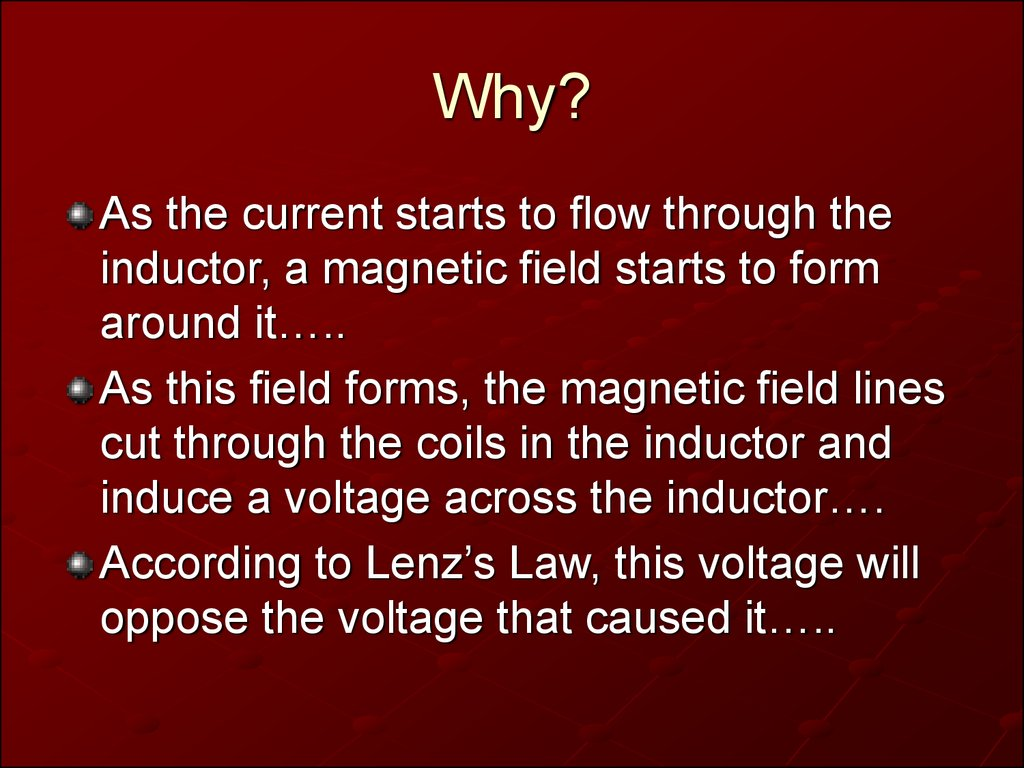# Inductors

## 1. Inductors

An inductor is essentially a coil of wire with an iron core.
They store energy in a magnetic field
The symbol for an inductor is:

## 2. What do inductors do?

Consider this circuit
What will happen
when the switch is
closed?
Lamp A will glow
immediately
Lamp B will take
some time to reach
full brightness.
A
B

## 3. Why?

As the current starts to flow through the
inductor, a magnetic field starts to form
around it…..
As this field forms, the magnetic field lines
cut through the coils in the inductor and
induce a voltage across the inductor….
According to Lenz’s Law, this voltage will
oppose the voltage that caused it…..

## 4. Why?

This opposing voltage restricts the current
build-up in the circuit so it takes a while for
the bulb to reach full brightness.
Current
building up
slowly
Opposing voltage (EMF)

## 5. Inductors

Once the current has reached it’s full
value, it is no longer changing, so the field
lines in the inductor are no longer building
up, so there is no induced back EMF. Ie
the inductor has no effect on the circuit
any longer.

## 6. Inductors

The reverse happens when the current is
switched off.
A collapsing current will cause the field
lines around the inductor to collapse.
The collapsing field lines induce a voltage
across the inductor to try and keep the
current flowing. (Lenz’s Law again)
This induced voltage can be very large,
creating sparks – dangerous!

## 7. Inductance

The effect of an inductor in a circuit is
measured as inductance L
The changing current produces a
changing field which produces a changing
flux ie Φ α I
The constant of proportionality is the
inductance L
So Φ = L x I

V
t
( L I )
V
t
I
V L
t

## 9. Inductance

So the size of the induced back EMF
depends on the inductance of the inductor
and the rate of change of current.
The unit for inductance is the Henry H
An inductor only affects circuits when the
current is changing and will always oppose
what is happening

## 10. Changing Current

When the switch is
closed, current starts
to flow in the circuit
It will build up to it’s
maximum value:
I max
V
R
V
A
L
R

I
I
Imax
Imax
t
t
Without Inductor
With Inductor

## 12. Time Constant

I
Imax
63%
t
t
The time constant t is
defined as the time
taken for the current
to reach 63% of it’s
maximum value.
A circuit is said to be
at maximum current
after 3 time constants
(Same as caps)

## 13. Time Constant

The time constant depends on:
The size of the inductor
(bigger L means more back
e.m.f so longer for current to
reach max)
The resistance of the circuit
(larger R means smaller
circuit current, so less mag
field, so less back e.m.f, so
shorter time for current to
reach max)
L
t
R

## 14. Back E.M.F

The back e.m.f will be
large to begin with as
the current is
changing most rapidly
It drops to zero as the
rate of change of
current reduces.
Again the time
constant is the time
taken to fall 63% of
max value
V
Vmax
t

## 15. Energy

Energy is stored in the magnetic field
around the inductor.
If the current is suddenly interupted,a
spark may occur as the energy is
dissipated. Self inductance can be a major
problem in big circuits because of this.
1
2
E LI
2

## 16. Transformers

These consist of 2
coils wound close to
each other.
Changing the current
in one coil makes the
field around it change.
This changing field
induces current in a
nearby coil.

## 17. Transformers

The ratio of the windings
determines how much
voltage/current is induced
The voltage can be
calculated using:
N=number of turns
V=Voltage
P=primary coil
S=secondary coil
Np
Ns
Vp
Vs

## 18. Transformers

No transformer is 100% efficient, but
assuming it was:
Power in = Power out
Pin Pout
V p I p Vs I s

## 19. Transformers

3 Types:
Step up : Vs > Vp
Step down : Vs < Vp
Isolating (a safety device) : Vs = Vp
They only work with alternating current or
switched DC